How to Show Gridlines on Matplotlib Plots?

• Last Updated : 28 Nov, 2021

In this article, we will see how we can add gridlines to a matplotlib graph and various configurable parameters that can be used in Python.

Example:

Create a matplotlib plot using sample data.

Python

 # Importing the libraryimport matplotlib.pyplot as plt  # Define X and Y data pointsX = [12, 34, 23, 45, 67, 89]Y = [1, 3, 67, 78, 7, 5]  # Plot the graph using matplotlibplt.plot(X, Y)  # Function to view the plotplt.show()

Output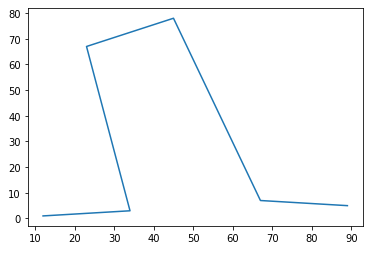grid()

The grid() method is used to define a grid in a matplotlib graph. The syntax is given by –

Syntax:

matplotlib.pyplot.grid(b=None, which=’major’, axis=’both’, **kwargs)

Parameters:

• b: bool or None, optional: Whether to show the grid lines. If any kwargs are supplied, it is assumed you want the grid on and b will be set to True. If b is None and there are no kwargs, this toggles the visibility of the lines.
• which: {‘major’, ‘minor’, ‘both’}, optional: The grid lines to apply the changes on.
• axis: {‘both’, ‘x’, ‘y’}, optional: The axis to apply the changes on.
• **kwargs: Line2D properties: Define the line properties of the grid

Example 1: Default Gridlines

Python

 # Importing the libraryimport matplotlib.pyplot as plt  # Define X and Y data pointsX = [12, 34, 23, 45, 67, 89]Y = [1, 3, 67, 78, 7, 5]  # Plot the graph using matplotlibplt.plot(X, Y)  # Add gridlines to the plotplt.grid(b=True)# `plt.grid()` also works  # Function to view the plotplt.show()

Output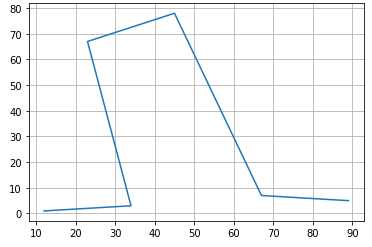Example 2: Apply Gridlines to  x- axis

In the cases, where we would want to see only the vertical or horizontal gridlines, we can make use of the `axis` parameter. To view only vertical gridlines –

Syntax:

plt.grid(b=True, axis='x')

Python3

 # Importing the libraryimport matplotlib.pyplot as plt  # Define X and Y data pointsX = [12, 34, 23, 45, 67, 89]Y = [1, 3, 67, 78, 7, 5]  # Plot the graph using matplotlibplt.plot(X, Y)  # Add gridlines to the plotplt.grid(b=True, axis='x')# `plt.grid()` also works  # Function to view the plotplt.show()

Output: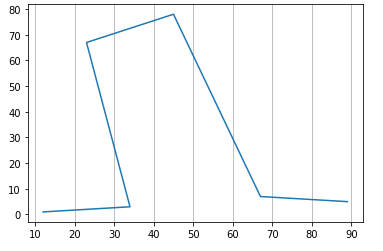Example 3: Apply Gridlines to y-axis

In the cases, where we would want to see only the vertical or horizontal gridlines, we can make use of the `axis` parameter. To view only horizontal gridlines –

Syntax:

plt.grid(b=True, axis='y')

Python3

 # Importing the libraryimport matplotlib.pyplot as plt  # Define X and Y data pointsX = [12, 34, 23, 45, 67, 89]Y = [1, 3, 67, 78, 7, 5]  # Plot the graph using matplotlibplt.plot(X, Y)  # Add gridlines to the plotplt.grid(b=True, axis='y')# `plt.grid()` also works  # Function to view the plotplt.show()

Output: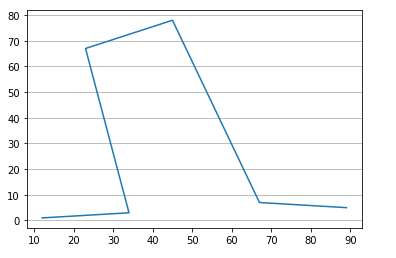Example 4: Provide a linestyle and linewidth

We have seen the gridlines being a solid lines. We can change this to view a different line-style like dashes using an argument `linestyle` or `ls`. Similarly, we can change the thickness of the gridlines using the argument `linewidth`. These arguments are provided as part of the keyword arguments and can be viewed in the document link.

To provide a line style –

Syntax:

plt.grid(linestyle='--')

Python3

 # Importing the libraryimport matplotlib.pyplot as plt  # Define X and Y data pointsX = [12, 34, 23, 45, 67, 89]Y = [1, 3, 67, 78, 7, 5]  # Plot the graph using matplotlibplt.plot(X, Y)  # Add gridlines to the plotplt.grid(linestyle='--')# `plt.grid()` also works  # Function to view the plotplt.show()

Output: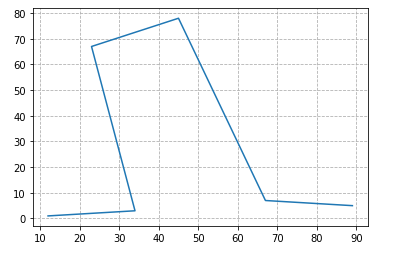.

Example 5: Change color of the Gridlines

The keyword argument `color` can be used to define a different color to the plot.

Syntax:

plt.grid(linestyle, color)

Python3

 # Importing the libraryimport matplotlib.pyplot as plt  # Define X and Y data pointsX = [12, 34, 23, 45, 67, 89]Y = [1, 3, 67, 78, 7, 5]  # Plot the graph using matplotlibplt.plot(X, Y)  # Add gridlines to the plotplt.grid(linestyle='--', color='pink')# `plt.grid()` also works  # Function to view the plotplt.show()

Output: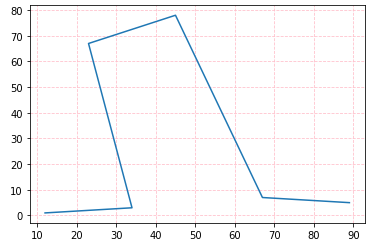My Personal Notes arrow_drop_up